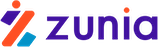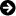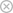Tuyển sinh 2023 dành cho Gen-Z# MULTIPLE LINEAR REGRESSION MODEL Introduction and Estimation

Chia sẻ: Hgiang Hgiang | Ngày: | Loại File: DOC | Số trang:12172
lượt xem
17

From the system we call the ‘normal equation system’ we can solve K normal equations for K unknown beta coefficients. The straight-forward representation of the solution is expressed in the matrix algebra. However, since the main purpose is the application and EViews. Other data analysis software is available, so we can easily find regression coefficients without remembering all the algebraic expressions.

Chủ đề:

## Nội dung Text: MULTIPLE LINEAR REGRESSION MODEL Introduction and Estimation

1. Fulbright Economics Teaching Program Analytical Methods Lecture notes 7 Lecture 7 MULTIPLE LINEAR REGRESSION MODEL Introduction and Estimation 1) Introduction to the multiple linear regression model The simple linear regression model cannot explain everything. So far, we have considered the simple linear regression model. In both theory and practice, there are many cases in which a given economic variable cannot be explained by such the simple regression model. We can offer the following examples : - Quantity demanded depends on price, income, and the prices of other goods, etc. Recall consumer behaviour theory. QD = f(P, I, Ps, Pc, Market size,Pf (expected price), T (preference)) Output depends on price, primary inputs, intermediate inputs, technology, etc. Recall production function theory : - QS=f(K,L, TECH) Nguyen Trong Hoai 1 3/29/09
2. Fulbright Economics Teaching Program Analytical Methods Lecture notes 7 the growth rate of an economy depends on investment, labour, technological change, etc. Recall the total factor productivity theory : - Wages depends on education, experience, gender, age, etc. House prices depends on size, the number of bedrooms and bathroom, etc. Nguyen Trong Hoai 2 3/29/09
3. Fulbright Economics Teaching Program Analytical Methods Lecture notes 7 Household expenditure on food depends on the household size, the income, the location, etc. National children mortality rates depends on the income per capita, eduction, etc. Nguyen Trong Hoai 3 3/29/09
4. Fulbright Economics Teaching Program Analytical Methods Lecture notes 7 The demand for money depends on the rate of interest, the price, the GDP in the economy; etc. When we collect data of some economic variables (called dependent variables) and its determinants (called explanatory variables), studies of separate influences (direct or net) of the various factors on an economic variable can be explained by the multiple regression model. 2) Data requirement Some data is expressed in terms of a spreadsheet as above mentioned. 3) Population Regression Function-PRF Study the model : - Yi = β 1 + β 2 X 2i + β 3 X 3i +  + β K X Ki + ε i PRF E[ Yi | X' s ] = β 1 + β 2 X 2i + β 3 X 3i +  + β K X Ki + E[ ε i | X' s ] The β -coefficients are called the partial regression coefficients and each one has the following interpretation : - Nguyen Trong Hoai 4 3/29/09
5. Fulbright Economics Teaching Program Analytical Methods Lecture notes 7 ∂ E[ Yi | X' s] = βk ∂X k 4) Important assumptions of the multiple linear regression model PRF consists of two components : a controlled part and a stochastic part (stochastic disturbance - random disturbance). ε i is a random variable and follows normal a distribution ε i ≈ N(0, σ 2), X’s are controlled variables or given variables. Since Yi is the sum of such two parts, Yi is also a random variable. 4.1 OLS assumptions in a simple regression model are interpreted in a multiple regression model : these assumptions relate to stochastic disturbance (ε i) a) Mean value of ε i is zero => E(ε i | X’s) = 0 b) No serial correlation (autocorrelation) => cov(ε i, ε j| X’s ) = 0 vôùi i # j c) Homoscedasticity => var(ε i) = σ 2 d) Random disturbance has no correlation with Xs => cov(ε i, Xki ) = 0 (k: number of explanatory variables in the model) e) No error model specification 4.2 Additional assumptions of OLS for multiple regression model Regressors do not perfectly satisfy any linear relationship (perfect multi- collinearity). That is, there is no set of coefficients for which the following expression is always true : - 1 + λ 2 X 2i + λ 3 X 3i +  + λ K X Ki = 0 We will explain this condition clearly by the two-explanatory variable (two regressor) model. We temporarily accept this assumption. 5) Sample Regression Function-SRF We address the estimation problem by specifying the sample regression function (SRF) : - Yˆi = βˆ1 + βˆ 2 X 2i + βˆ 3 X 3i +  + βˆ K X Ki The residuals are defined in just the same way as they were defined in the simple regression framework : - ˆ ei = Yi - Yi Nguyen Trong Hoai 5 3/29/09
6. Fulbright Economics Teaching Program Analytical Methods Lecture notes 7 6) Ordinary Least Squares Estimators - OLS By definition, we can invoke the ordinary least squares principle to choose the estimators of partial regression coefficients. ˆ ˆ ˆ Choose β 1 , β 2 ,  , β K to minimize ∑e 2 i . ∑e ∑( Y ) 2 Note that 2 = ˆ ˆ ˆ ˆ - β 1 - β 2 X 2i - β 3 X 3i -  - β K X Ki i i We can set the first-order conditions of the minimization exercise as : - ∂ ∑ ei2 ˆ ( = - 2∑ Yi - β 1 - β 2 X 2i - β 3 X 3i -  - β K X Ki ˆ ˆ ˆ ˆ )=0 ∂β 1 ∂ ∑ ei2 ˆ ( = - 2∑ Yi - β 1 - β 2 X 2i - β 3 X 3i -  - β K X Ki X 2i = 0 ˆ ˆ ˆ ˆ ) ∂β 2  ∂ ∑ ei2 ˆ ( = - 2∑ Yi - β 1 - β 2 X 2i - β 3 X 3i -  - β K X Ki X Ki = 0 ˆ ˆ ˆ ˆ ) ∂β K From the system we call the ‘normal equation system’ we can solve K normal equations for K unknown beta coefficients. The straight-forward representation of the solution is expressed in the matrix algebra. However, since the main purpose is the application and EViews. Other data analysis software is available, so we can easily find regression coefficients without remembering all the algebraic expressions. 7) The Two Explanatory Variable (two regressor) Regression Model We can present a solution for the model that contains two regressors : - Yi = β 1 + β 2 X 2i + β 3 X 3i + ε i First, we must write down a normal equation system for the case, then use matrix algebra to find the estimators. The least-squares estimators are : - βˆ1 = Y - βˆ 2 X 2 - βˆ 3 X 3 Nguyen Trong Hoai 6 3/29/09
7. Fulbright Economics Teaching Program Analytical Methods Lecture notes 7 (∑ y x ) (∑ x ) - (∑ y x ) (∑ x x ) 2 βˆ 2 = i 2i 3i i 3i 2i 3i (∑ x ) (∑ x ) - (∑ x x ) 2 2i 2 3i 2i 3i 2 (∑ y x ) (∑ x ) - (∑ y x ) (∑ x x ) 2 βˆ 3 = i 3i 2i i 2i 2i 3i (∑ x ) (∑ x ) - (∑ x x ) 2 2i 2 3i 2i 3i 2 We do not need to remember these expressions, but we will use them to demonstrate certain results. The calculation of the estimators will become more difficult if our regression model has more regressors. However, with the help of Eviews and other data analysis software, we can find the estimators of the multiple regression model quickly and easily. To explain when there is perfect multi-collinearity, we cannot receive finite solutions for the regression coefficients. 8) Meaning of estimated coefficients in the multiple regression model Name : partial slope coefficient or partial regression coefficient. Meaning : the partial slope coefficient of regression variables in the multiple regression model describes by how many units the dependent variable changes when the explanatory variable changes by one unit - holding other explanatory variables constant. In other words, the partial slope coefficient reflects the net effect or the direct effect of the dependent variable when the explanatory variable changes by one unit – and after having removed the influences of any other regression variables. The effectiveness of multiple regression model : it directly estimates the direct effect of the one regression variable on the dependent variable. If we use a multiple regression model to estimate the direct effect of one regression variable on the dependent variable (for example, where the one dependent variable depends upon two regression variables X2 and X3). If we want to find out the direct effect of X2 on the dependent variable (Y in this case), we must do three simple regressions. For example : we have data on the child mortality rate (CM) which depends on the GNP per capita (PGNP) and the female illiteracy rate (FLR). If we want to find out the direct effect of PGNP on CM, we remove the effect of FLR on CM and PGNP. Please see the example in the Reading, pages 206 and 214 (English version). - Regress CM on FLR = CMi = 263.8635 – 2.3905.FLRi + e1i Nguyen Trong Hoai 7 3/29/09
8. Fulbright Economics Teaching Program Analytical Methods Lecture notes 7 - - Regress PGNP on FLR = PGNP = - 39.3033 + 28.1427 FLRi + e2i - Nguyen Trong Hoai 8 3/29/09
9. Fulbright Economics Teaching Program Analytical Methods Lecture notes 7 - Regress the resedual of the first function on the resedual of the second equation = e1i^= -0.0056 e2i - Multiple regression helps us immediately to know the direct effect of the PGNP on the CM with the same value as is calculated in the third simple regression. CM^ = 263.6416 – 0.0056 PGNPi – 2.2326 FLRi Nguyen Trong Hoai 9 3/29/09
10. Fulbright Economics Teaching Program Analytical Methods Lecture notes 7 We can explain more by using a graph. 9) The Variance (VAR) and standard deviation (SE) of the estinators SE (estimated) = root square of VAR (estimated) Variances of multiple regression are also very complicated. We will only write ˆ down the variance of β 2 to see this as an example : - ˆ VAR β 2 =[ ] ∑x 2 3i σ2 (∑ x ) (∑ x ) - (∑ x 2 2i 2 3i x 2i 3i ) 2 Recall the definition of the squared correlation coefficient between X2 and X3 : - (∑ x x ) 2i 3i 2 r23 = 2 (∑ x ) (∑ x ) 2 2i 2 3i ˆ By manipulating a little bit we can rewrite the variance of β 2 as follows : - 1 VAR  βˆ2  = σ2=σ2   ( ∑ x ) (1 - r ) ∧ 2 2 β K=2 2i 23 Again, if these two regressors are uncorrected, then the variance is simplified to it’s simple regression counterpart. Sampling probability distributions of OLS estimators In order to be able to construct intervals with confidence for the unknown parameters, and to best test their hypotheses, we need to know the sampling probability distributions for the estimators. When we mention the sampling distribution, we require three things : - 1. The mathematical expectation 2. The variance 3. The functional form ˆ First consider the expectation of an estimator β 2 : - Nguyen Trong Hoai 10 3/29/09
11. Fulbright Economics Teaching Program Analytical Methods Lecture notes 7 (∑ Y x ) (∑ x ) - (∑ Y x ) (∑ x x ) 2 βˆ 2 = i 2i 3i i 3i 2i 3i (∑ x ) (∑ x ) - (∑ x x ) 2 2i 2 3i 2i 3i 2 Now substitute : - Yi = β 1 + β 2 X 2i + β 3 X 3i + ε i into the expression and make some changes algebraically : - ˆ (∑ Y x ) (∑ x ) - (∑ Y x ) (∑ x i 2i 2 3i i 3i 2i x 3i ) β2 = (∑ x ) (∑ x ) - (∑ x x )2 2i 2 3i 2i 3i 2 (∑ ε x ) (∑ x ) - (∑ ε x ) (∑ x i 2i 2 3i i 3i 2i x 3i ) = β2 + (∑ x ) (∑ x ) - (∑ x x ) 2 2i 2 3i 2i 3i 2 When we take the expectation of the second expression, we find that our estimator is unbiased because of the following results : - [ ˆ E β2 ]=β 2 We are already familiar with the variance. Finally, it is apparent from the expression above that each estimator is a linear combination of normally distributed random variables, so each estimator is also normally distributed. The same results are true for the K-variable multiple regression. The estimators are unbiased, their variances are known, and they are normally distributed. However, these results are impractical to demonstrate without matrix algebra. We summarize the typical result this way : - ( βˆk ~ N β k , σ β2ˆ k ) 10) Properties of OLS estimators in the multiple regression model 10.1 BLUE – “Best Linear Unbiased Estimator.” This property is the same as for the simple regression model. We should understand three properties of BLUEø : - Nguyen Trong Hoai 11 3/29/09
12. Fulbright Economics Teaching Program Analytical Methods Lecture notes 7 1. Linear estimators (linear regression coefficients - give some examples) 2. Unbiased estimators (based on the estimation expression - we can get expectation of both sides). 3. Variance of estimators is minimum (it is proved by Gauss-Markov, however, we can interpret the estimators with a minimum variance directly when referring to the covariance of regression variables - and assuming that there is no perfect collinearity). 10.2 When there is perfect multi-collinearity (i.e. do not satisfy the OLS assumptions for the multiple regression model), the VAR of the estimated coefficients is not minimized and we cannot find the estimators for the coefficients. 10.3 The more the change in the regression variable in comparison with its mean, the less the variance of the estimated coefficient, and the more accurate the estimated parameters becomes. It is normal that the more the change in the regression variable, the more accurate the sample size (number of observations) becomes. This can be explained using a probability density function graph. So, what can be said to be a large enough sample size? Nguyen Trong Hoai 12 3/29/09